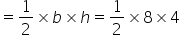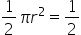Maths-
General
Easy

Question

# The largest triangle is inscribed in a semi-circle of radius 4 cm. Find the area inside the semi-circle which is not occupied by the triangle.Hint:

## The correct answer is: 9.12 cm2

### It is given that radius of the triangle = 4 cmBase of the triangle = 2r = 8 cmHeight of the triangle = r = 4 cmArea of the triangle == 16 cm2Area of semi-circle ==   (3.14) 42= 25.12 cm2Area not occupied by the triangle= Area of semi-circle – Area of triangle= 25.12 – 16 = 9.12 cm2#### With Turito Foundation.#### Get an Expert Advice From Turito.